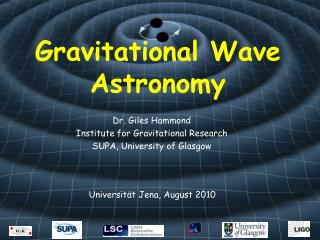DownloadDownload PresentationGravitational Wave Astronomy

# Gravitational Wave Astronomy

Télécharger la présentation## Gravitational Wave Astronomy

- - - - - - - - - - - - - - - - - - - - - - - - - - - E N D - - - - - - - - - - - - - - - - - - - - - - - - - - -
##### Presentation Transcript

1. Gravitational Wave Astronomy Dr. Giles Hammond Institute for Gravitational Research SUPA, University of Glasgow Universität Jena, August 2010

2. Mathematical Tools of GR • The spacetime of gravity is described by a Riemann metric • A continuous space which can be differentiated everywhere and is locally flat • In GR we need to define how vectors and their basis sets transform in this space.

3. y x Mathematical Tools of GR • A Riemann space can be differentiated everywhere • Point X has coordinates • Can define the gradient of a function  at X as the space can be differentiated • Can think of as a set of basis vectors which span the space • In general the basis at X and Y will be different => given a coordinate system we can construct a natural basis for a vector field

4. Vectors and One-Forms • It is necessary to introduce briefly vectors and one-forms • Consider a 2-D rotation • Transformation rule for a vector (denoted by superscript):

5. Vectors and One-Forms • Now consider a scalar field (r) : How does  transform under a rotation? • For example: • Transformation rule for a one-form (denoted by subscript):

6. Vectors and One-Forms • We can show that • In 2-D Euclidean space the same results are obtained • This is not the case in general spaces (e.g change of basis from Cartesian to Polar coordinates) • Any vector space can be written in terms of a dual-basis (covariant/contravariant). The metric tensor maps between them. vector one-form

7. Parallel Transport • Differentiation of a vector field involves subtracting vector components at different points (i.e. P and Q) • Need a procedure which parallel translates components of A to point Q • This is called Covariant Differentiation • where • are the Christoffel symbols which are used to connect the basis vectors at Q to those at P (i.e. stitch together spacetime)

8. Christoffel Symbols • The Christoffel symbols can be written in terms of the metric • and thus we can define how vector fields transport over the surface of a general curved space • A geodesic in spacetime is a curve along which the tangent vector is parallel translated. Thus it also includes the Christoffel symbols • Material particles under the influence of gravity follow geodesics

9. Curvature II • The curvature of gravitational spacetime is described by the Riemann tensor => components can be obtained by parallel translation of a vector Flat space ( = 0) Curved space ( 0) • The Riemann tensor provides the mathematical tool to describe curvature • It is fairly simple to write programs which calculate the components of the Riemann tensor for general spaces

10. Simple Example • Consider polar coordinates dr and d. The line element is • The only non-zero Christoffel symbols are dr r ds d

11. Simple Example • All the components of the Riemann tensor are zero => Flat space. The 1st term for example is • And the geodesic equations are • The equations of motion can also be derived from the Lagrangian of the interval

12. More Complicated Examples • There are add-on packages to Mathematica which turn the program into a powerful symbolic manipulator of tensors • http://www.inp.demokritos.gr/~sbonano/RGTC/ • Eg. 1 The polar coordinate space already examined => Flat space with all components of the Riemann tensor=0 • Eg 2. The surface of a 2-D sphere defined by polar coordinates  and  => Curved space (constant curvature) with some non-zero components of the Riemann tensor

13. Ricci Tensor/Scalar • The Riemann tensor in 4-D has 256 components (20 independent components) • We can produce a 2-index tensor (16 components) by contraction. This gives the Ricci tensor; • And the Ricci scalar or curvature scalar is • The Ricci scalar of a sphere, radius a, is